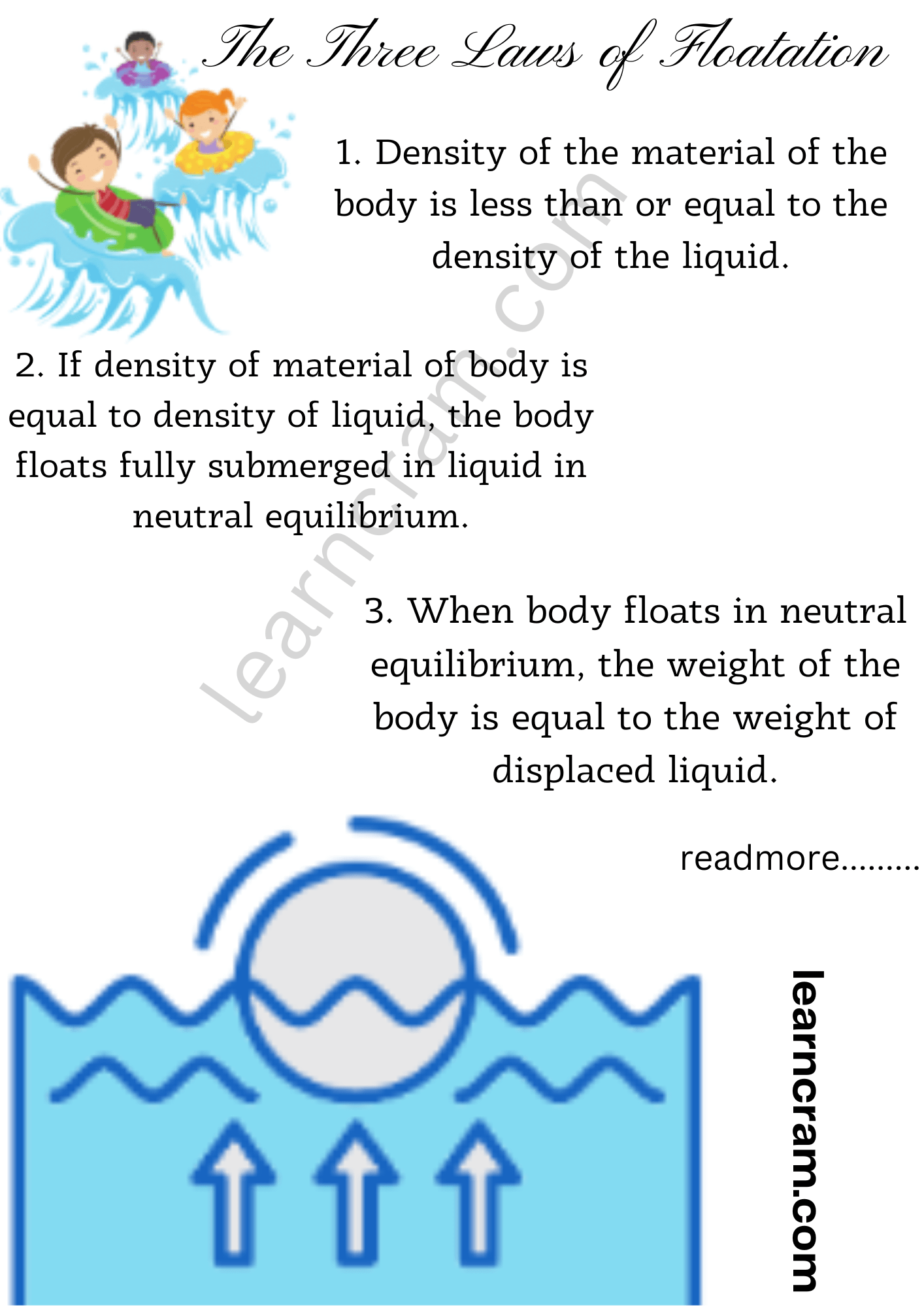# State the Law of Floatation in Physics – Hydrostatics

Law of Floatation in Physics:
A body will float in a liquid, if the weight of the body is equal to the weight of the liquid displaced by the immersed part of the body.

We are giving a detailed and clear sheet on all Physics Notes that are very useful to understand the Basic Physics Concepts.

## State the Law of Floatation in Physics – Hydrostatics

The Three Laws of Floatation:
1. Density of the material of the body is less than or equal to the density of the liquid.
2. If density of material of body is equal to density of liquid, the body floats fully submerged in liquid in neutral equilibrium.
3. When body floats in neutral equilibrium, the weight of the body is equal to the weight of displaced liquid.

If W is the weight of the body and w is the buoyant force, then

1. If W > w, then body will sink to the bottom of the liquid.
2. If W < w, then body will float partially submerged in the liquid.
3. If W = w, then body will float in liquid if its whole volume is just immersed in the liquid.

The floating body will be in stable equilibrium, if meta-centre (centre of buoyancy) lies vertically above the centre of gravity of the body.

The floating body will be in unstable equilibrium, if meta-centre (centre of buoyancy) lies vertically below the centre of gravity of the body. The floating body will be in neutral equilibrium, if meta-centre (centre of buoyancy) coincides with the centre of gravity of the body.

Fraction of volume of a floating body outside the liquid

$$\left(\frac{V_{\text {out }}}{V}\right)=\left[1-\frac{\rho}{\sigma}\right]$$

where,
ρ = density of body
σ = density of liquid

If two different bodies A and B are floating in the same liquid, then

$$\frac{\rho_{A}}{\rho_{B}}=\frac{\left(v_{\text {in }}\right)_{A}}{\left(v_{\text {in }}\right)_{B}}$$

If the same body is made to float in different liquids of densities σA and σB respectively, then

$$\frac{\sigma_{A}}{\sigma_{B}}=\frac{\left(V_{\text {in }}\right)_{B}}{\left(V_{\text {in }}\right)_{A}}$$Hydrostatics:
Hydrostatics is a property of liquid or fluid in mechanics. A fluid is a material which flows at room temperature, because its upper molecule overlaps the inner molecule, which tends to flow the liquid in forward direction. In hydrostatics, we will learn about the condition of fluids when it is in rest or exerted by an external force. Here we will study the fluids in motion.